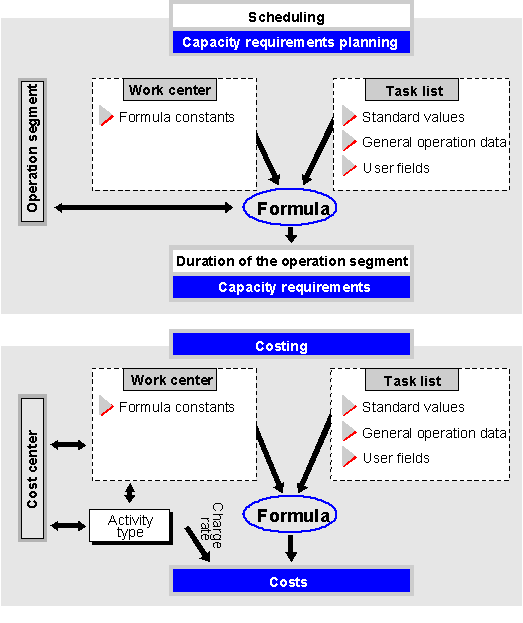Show TOC

###FormulasYou use formulas in the work center to calculate the following data in costing, scheduling and capacity planning:

• Costs

• Execution times for production orders

• Internal processing times for networks and maintenance orders

• Capacity requirements.

In the SAP system you can:

• use predefined SAP formulas

You can create formulas in Customizing Work centers under Define formulas for work centers .

#### Relationships between work centers, routings and formulas

The following graphic illustrates the relationships between work centers, routings and formulas for costing, scheduling and capacity requirements calculation. Formulas are entered in the work center; they take their values from the work center (formula constants) and the routing (standard values, general operation data and user-defined fields.Relationships between work centers, routings and formulas

#### Costs

In production orders each of up to six standard values in a work center can be assigned an activity type and a formula with which the costs of the operations conducted in the work center are calculated.

For networks and service orders an activity type and formula can be defined.

#### Execution Time

You use formulas to calculate execution time and consequently the duration of operations, which you need to schedule production orders

You can calculate the execution time for each operation segment separately (setting up, processing and tearing down) by assigning a formula to each of these segments. The execution time for the whole operation is the sum of the execution times of the individual segments. You can however just assign a formula to one segment (for instance processing), in which you take into account the durations of the other segments.

If you do not assign a formula to an operation segment, the execution time of this segment will be set to zero.

The program checks that the formula has the dimension “time”.

#### Internal Processing Time

Formulas are used to determine internal processing time for scheduling networks and maintenance orders, for example. If you do not enter a formula, the system will use the time given in the network or maintenance order.

The program checks that the formula has the dimension “time”.

#### Capacity Requirements

You can assign a formula to each operation segment (setting up, processing and tearing down) to calculate the capacity requirements. The capacity requirements for the whole operation are then calculated from the individual segments. If you do not assign a formula to an operation segment, the execution time of this segment will be set to zero.

You can also use a formula to calculate capacity requirements for internal processing in a network or a service order.

If you do not enter a formula, no capacity requirements will be determined.